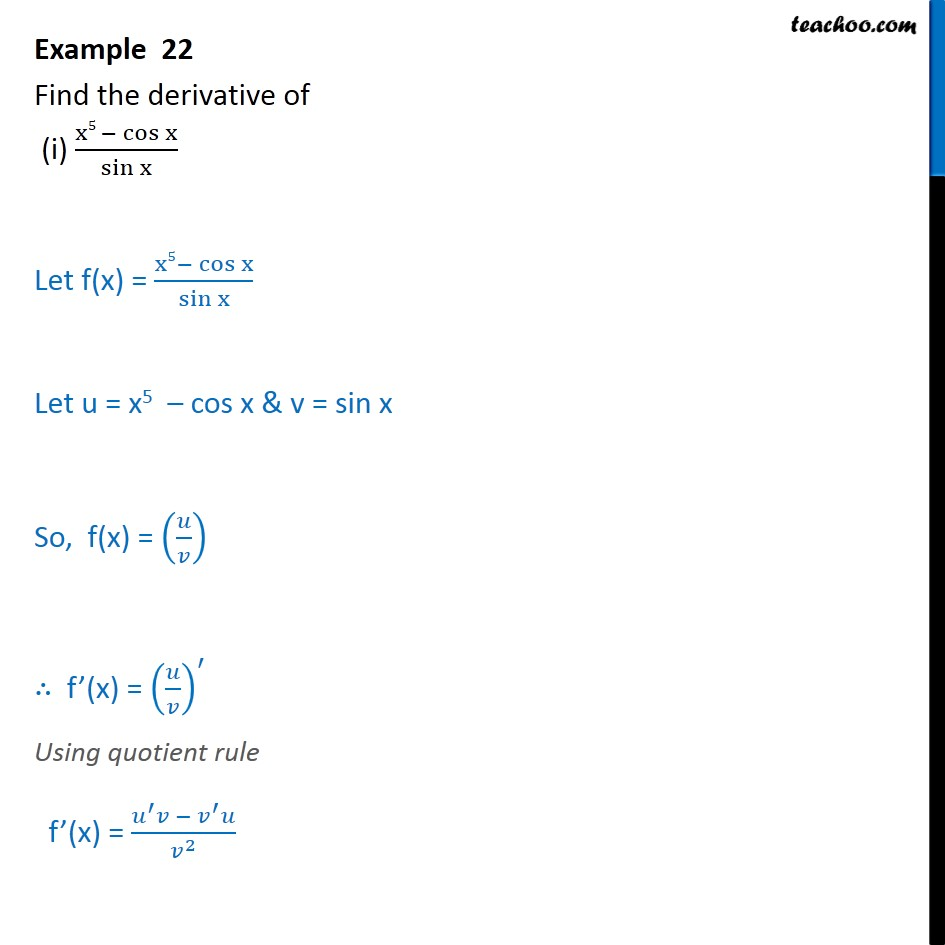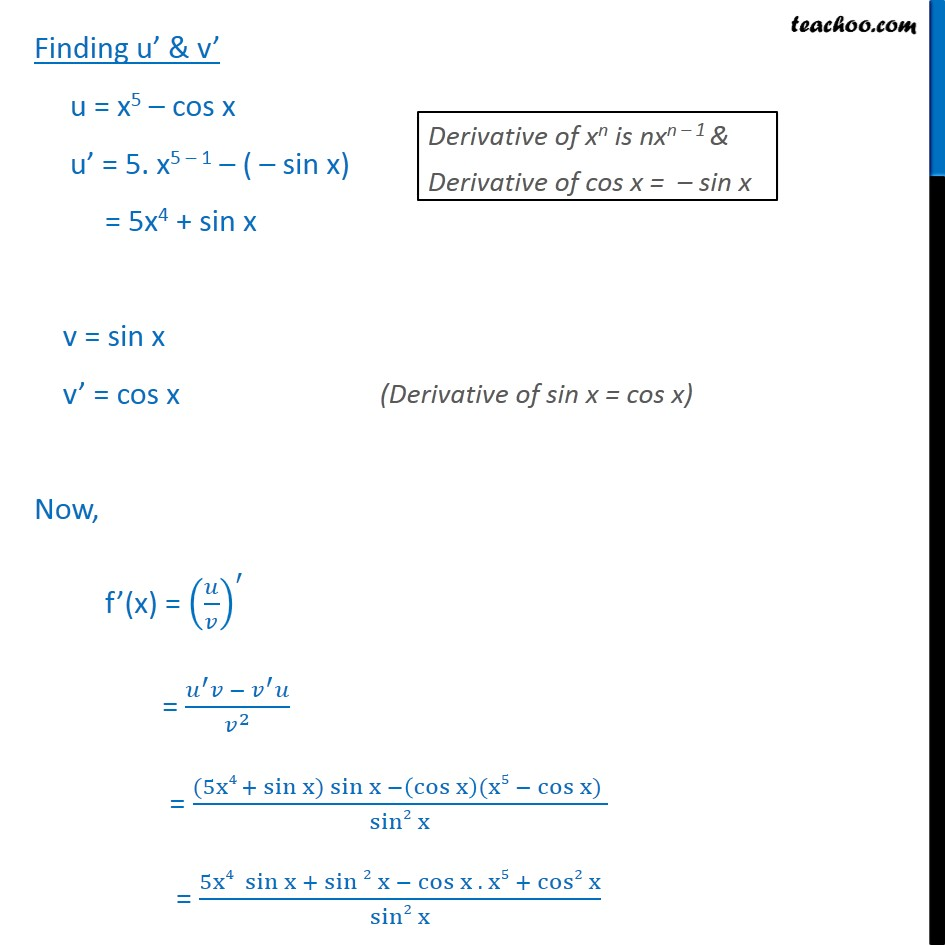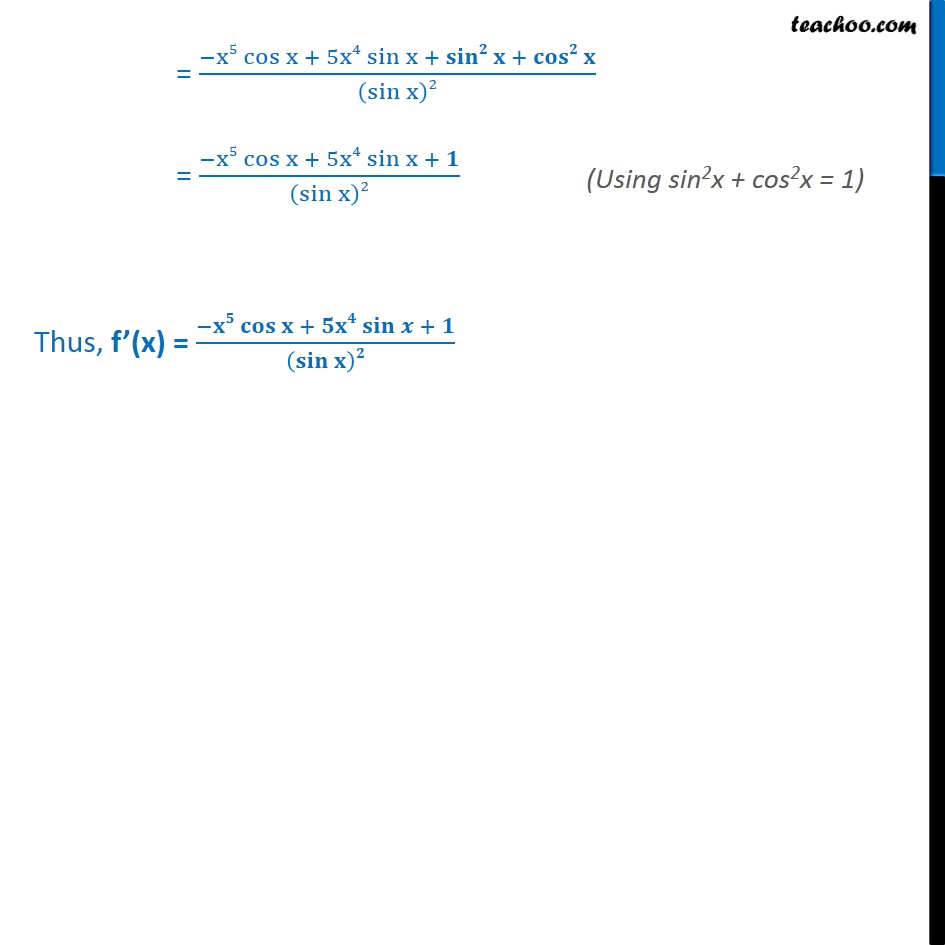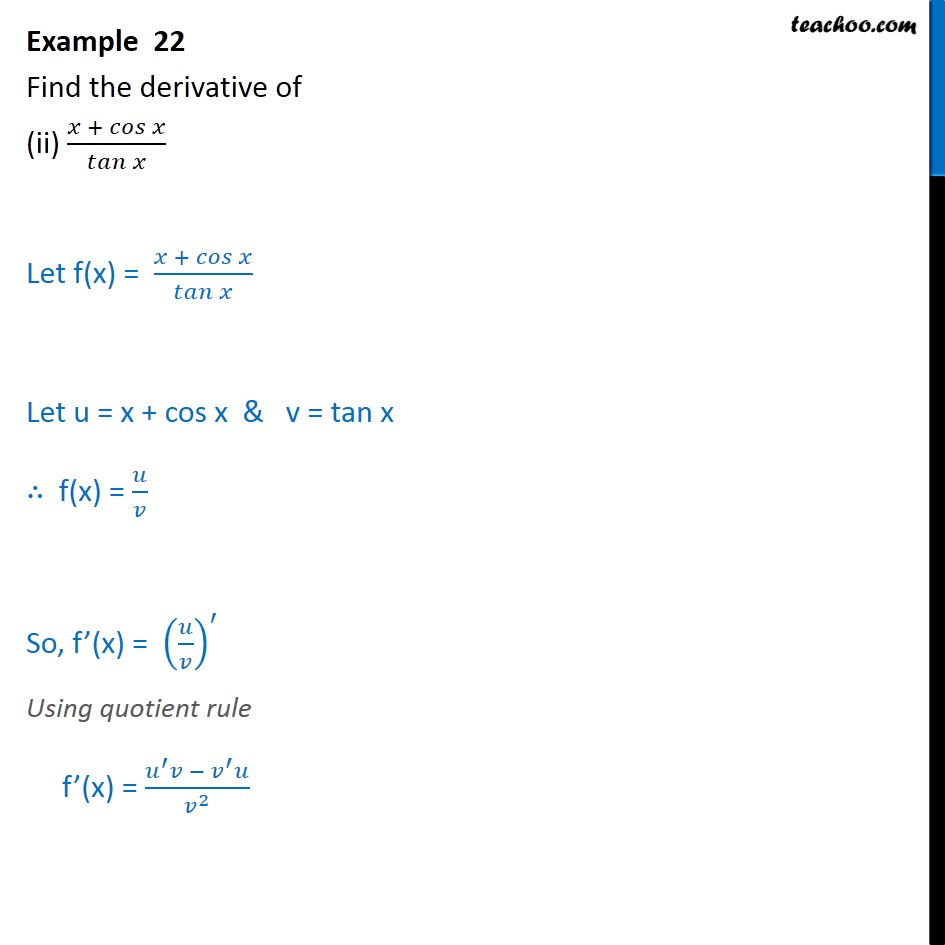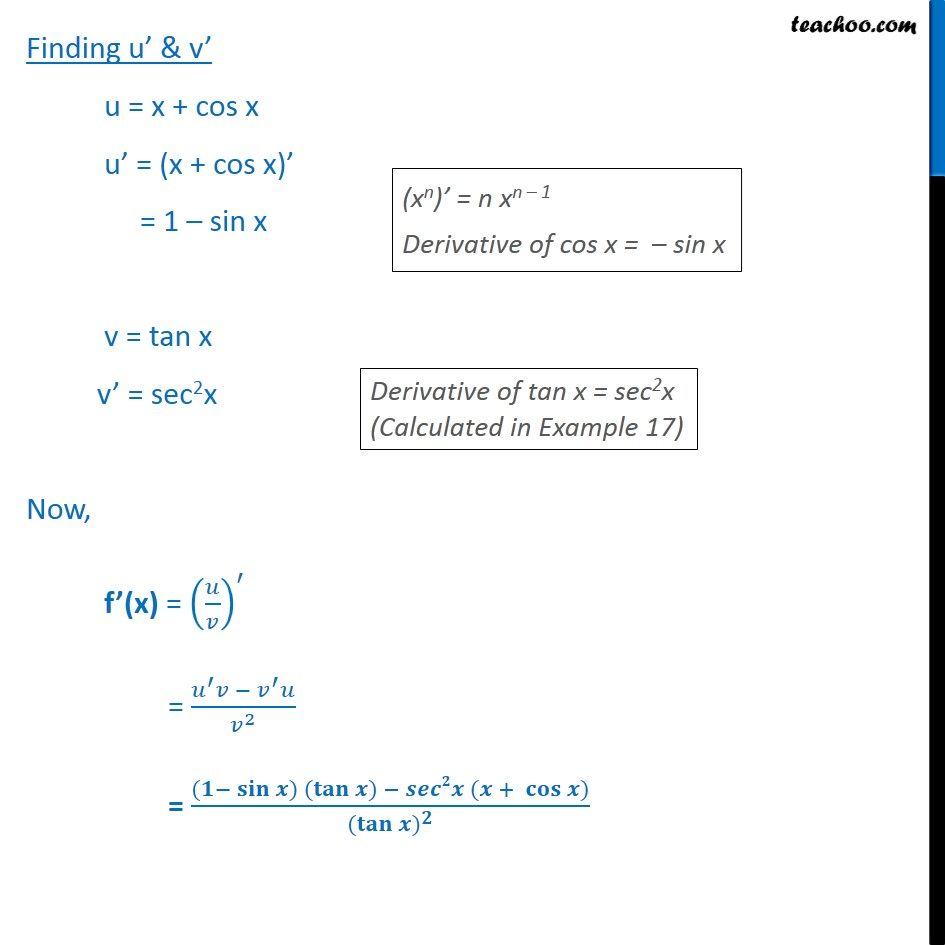1. Class 11
2. Important Question for exams Class 11
3. Chapter 13 Class 11 Limits and Derivatives

Transcript

Example 22 Find the derivative of (i) x5 − cos﷮x﷯﷮ sin﷮x﷯﷯ Let f(x) = x5− cos﷮x﷯﷮ sin﷮x﷯﷯ Let u = x5 – cos x & v = sin x So, f(x) = 𝑢﷮𝑣﷯﷯ ∴ f’(x) = 𝑢﷮𝑣﷯﷯﷮′﷯ Using quotient rule f’(x) = 𝑢﷮′﷯𝑣 − 𝑣﷮′﷯𝑢﷮ 𝑣﷮2﷯﷯ Finding u’ & v’ u = x5 – cos x u’ = 5. x5 – 1 – ( – sin x) = 5x4 + sin x v = sin x v’ = cos x Now, f’(x) = 𝑢﷮𝑣﷯﷯﷮′﷯ = 𝑢﷮′﷯𝑣 − 𝑣﷮′﷯𝑢﷮ 𝑣﷮2﷯﷯ = (5x4 + sin﷮x) sin x − cos x﷯(x5 − cos x) ﷯﷮ sin2﷮x﷯﷯ = 5x4 sin﷮x + sin 2﷮x﷯ − cos x . x5 + cos2 x﷯﷮sin2 x﷯ = −x5 cos﷮x + 5x4 sin﷮x﷯ + 𝐬𝐢𝐧𝟐 𝐱 + 𝐜𝐨𝐬𝟐 𝐱﷯﷮ sin﷮x﷯﷯2﷯ = −x5 cos﷮x + 5x4 sin﷮x﷯ + 𝟏﷯﷮ sin﷮x﷯﷯2﷯ Thus, f’(x) = −𝐱𝟓 𝐜𝐨𝐬﷮𝐱 + 𝟓𝐱𝟒 𝐬𝐢𝐧﷮𝒙﷯ + 𝟏﷯﷮ 𝐬𝐢𝐧﷮𝐱﷯﷯𝟐﷯ Example 22 Find the derivative of (ii) 𝑥 + 𝑐𝑜𝑠﷮𝑥﷯﷮ 𝑡𝑎𝑛﷮𝑥﷯﷯ Let f(x) = 𝑥 + 𝑐𝑜𝑠﷮𝑥﷯﷮ 𝑡𝑎𝑛﷮𝑥﷯﷯ Let u = x + cos x & v = tan x ∴ f(x) = 𝑢﷮𝑣﷯ So, f’(x) = 𝑢﷮𝑣﷯﷯﷮′﷯ Using quotient rule f’(x) = 𝑢﷮′﷯𝑣 − 𝑣﷮′﷯𝑢﷮ 𝑣﷮2﷯﷯ Finding u’ & v’ u = x + cos x u’ = (x + cos x)’ = 1 – sin x v = tan x v’ = sec2x Now, f’(x) = 𝑢﷮𝑣﷯﷯﷮′﷯ = 𝑢﷮′﷯𝑣 − 𝑣﷮′﷯𝑢﷮ 𝑣﷮2﷯﷯ = (𝟏− 𝐬𝐢𝐧﷮𝒙) ( 𝐭𝐚𝐧﷮𝒙) − 𝒔𝒆𝒄𝟐𝒙 (𝒙 + 𝐜𝐨𝐬﷮𝒙)﷯﷯﷯﷮ ( 𝐭𝐚𝐧﷮𝒙)﷯﷮𝟐﷯﷯

Chapter 13 Class 11 Limits and Derivatives

Class 11
Important Question for exams Class 11

About the AuthorDavneet Singh
Davneet Singh is a graduate from Indian Institute of Technology, Kanpur. He has been teaching from the past 9 years. He provides courses for Maths and Science at Teachoo.